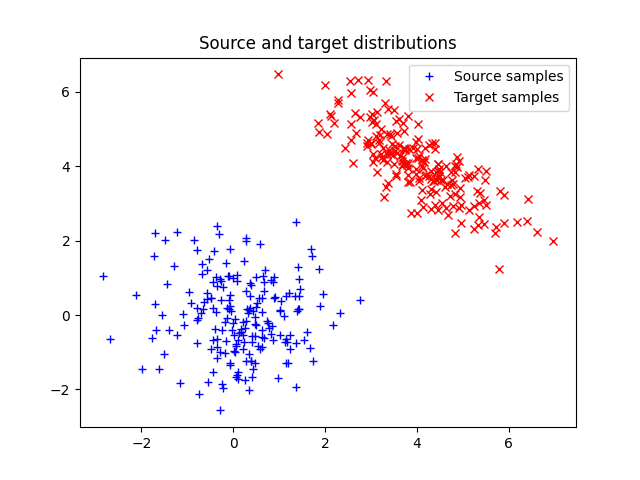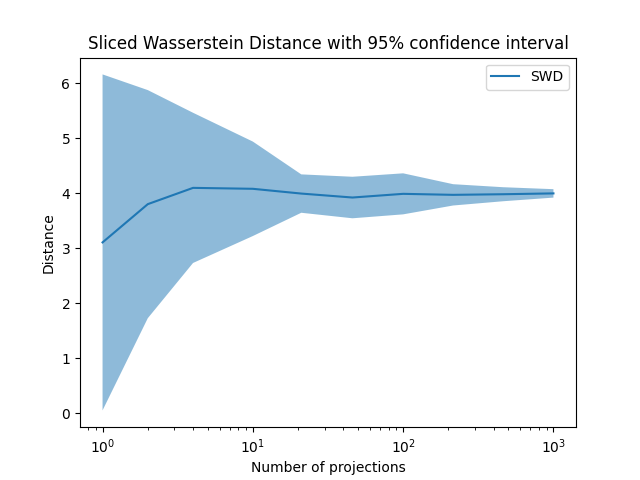# Sliced Wasserstein Distance on 2D distributions

This example illustrates the computation of the sliced Wasserstein Distance as proposed in .

 Bonneel, Nicolas, et al. “Sliced and radon wasserstein barycenters of measures.” Journal of Mathematical Imaging and Vision 51.1 (2015): 22-45

```# Author: Adrien Corenflos <adrien.corenflos@aalto.fi>
#

# sphinx_gallery_thumbnail_number = 2

import matplotlib.pylab as pl
import numpy as np

import ot
```

## Generate data

```n = 500  # nb samples

mu_s = np.array([0, 0])
cov_s = np.array([[1, 0], [0, 1]])

mu_t = np.array([4, 4])
cov_t = np.array([[1, -.8], [-.8, 1]])

xs = ot.datasets.make_2D_samples_gauss(n, mu_s, cov_s)
xt = ot.datasets.make_2D_samples_gauss(n, mu_t, cov_t)

a, b = np.ones((n,)) / n, np.ones((n,)) / n  # uniform distribution on samples
```

## Plot data

```pl.figure(1)
pl.plot(xs[:, 0], xs[:, 1], '+b', label='Source samples')
pl.plot(xt[:, 0], xt[:, 1], 'xr', label='Target samples')
pl.legend(loc=0)
pl.title('Source and target distributions')
```Out:

```Text(0.5, 1.0, 'Source and target distributions')
```

## Sliced Wasserstein distance for different seeds and number of projections

```n_seed = 50
n_projections_arr = np.logspace(0, 3, 25, dtype=int)
res = np.empty((n_seed, 25))
```
```for seed in range(n_seed):
for i, n_projections in enumerate(n_projections_arr):
res[seed, i] = ot.sliced_wasserstein_distance(xs, xt, a, b, n_projections, seed=seed)

res_mean = np.mean(res, axis=0)
res_std = np.std(res, axis=0)
```

## Plot Sliced Wasserstein Distance

```pl.figure(2)
pl.plot(n_projections_arr, res_mean, label="SWD")
pl.fill_between(n_projections_arr, res_mean - 2 * res_std, res_mean + 2 * res_std, alpha=0.5)

pl.legend()
pl.xscale('log')

pl.xlabel("Number of projections")
pl.ylabel("Distance")
pl.title('Sliced Wasserstein Distance with 95% confidence inverval')

pl.show()
```Total running time of the script: ( 0 minutes 46.109 seconds)

Gallery generated by Sphinx-Gallery